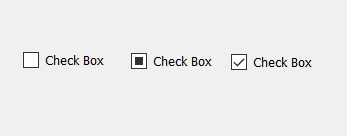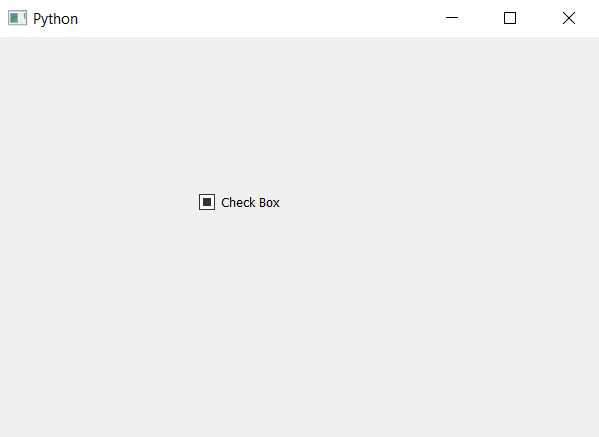# PyQt5 – Tri-state Check Box

• Last Updated : 22 Apr, 2020

When we create a check box, by default there are two state which are either checked i.e True and other is False. But we can add third state to it which is neither True or False with the help of `setTristate` method.

Below is how all three states looks like.Syntax : checkbox.setTristate(True)

Argument : It takes bool as argument.

Action performed : It will add third state to the check box.

Below is the implementation.

 `# importing libraries``from` `PyQt5.QtWidgets ``import` `*` `from` `PyQt5 ``import` `QtCore, QtGui``from` `PyQt5.QtGui ``import` `*` `from` `PyQt5.QtCore ``import` `*` `import` `sys`` ` ` ` `class` `Window(QMainWindow):`` ` `    ``def` `__init__(``self``):``        ``super``().__init__()`` ` `        ``# setting title``        ``self``.setWindowTitle(``"Python "``)`` ` `        ``# setting geometry``        ``self``.setGeometry(``100``, ``100``, ``600``, ``400``)`` ` `        ``# calling method``        ``self``.UiComponents()`` ` `        ``# showing all the widgets``        ``self``.show()`` ` `    ``# method for widgets``    ``def` `UiComponents(``self``):`` ` `        ``# creating the check-box``        ``checkbox ``=` `QCheckBox(``'Check Box'``, ``self``)`` ` `        ``# setting geometry of check box``        ``checkbox.setGeometry(``200``, ``150``, ``100``, ``30``)`` ` `        ``# creating the third state``        ``checkbox.setTristate(``True``)`` ` ` ` `# create pyqt5 app``App ``=` `QApplication(sys.argv)`` ` `# create the instance of our Window``window ``=` `Window()`` ` `# start the app``sys.exit(App.``exec``())`

Output :My Personal Notes arrow_drop_up